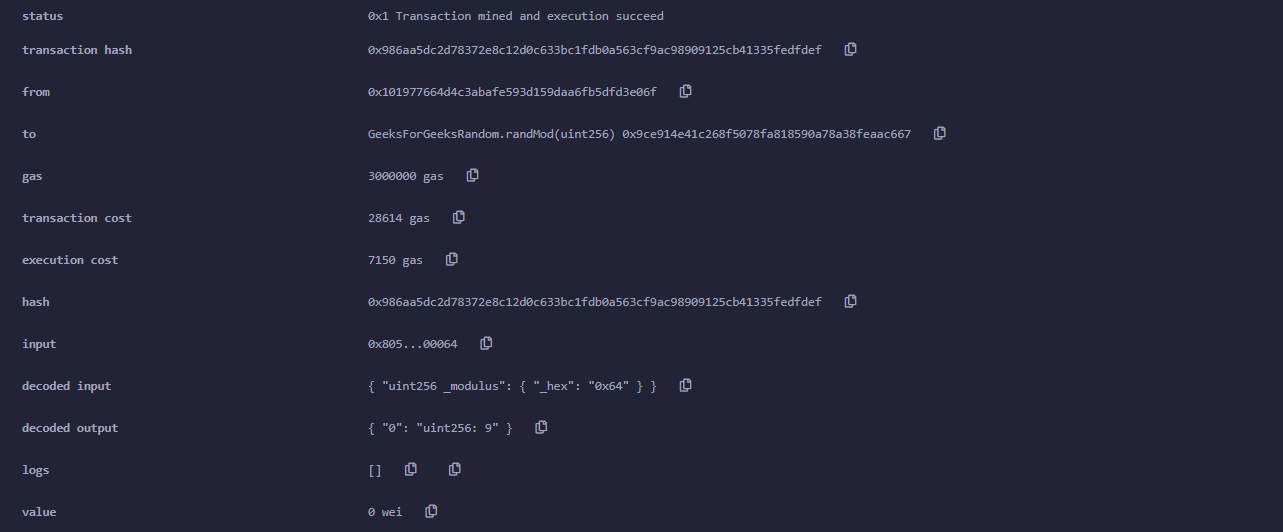# Random Number Generator in Solidity using keccak256

• Difficulty Level : Medium
• Last Updated : 11 May, 2022

Random numbers are numbers that occur in a sequence with the following two mandatory conditions:

1. The values are uniformly distributed over a set interval.
2. One cannot predict future values based on past outputs.

Example: In the below example, we have created a contract with a function that will generate a random number. Below is the step by step description of the entire process to be followed.

Step 1: Take the instance of now, the msg.sender, and an incrementing nonce.

Step 2: “pack” the inputs and use keccak256() to convert into 256-bit hash.

Step 3: Convert that hash to an uint, and then use % 100 to take only the last 2 digits. This will give us a totally random number between 0 and 99.

## Solidity

 `// Solidity program to` `// demonstrate on how ` `// to generate a random number` `pragma solidity ^0.6.6;`   `// Creating a contract` `contract` `GeeksForGeeksRandom ` `{`   `// Initializing the state variable` `uint randNonce = 0;`   `// Defining a function to generate` `// a random number` `function randMod(uint _modulus) internal returns(uint) ` `{` `   ``// increase nonce` `   ``randNonce++;  ` `   ``return` `uint(keccak256(abi.encodePacked(now, ` `                                          ``msg.sender, ` `                                          ``randNonce))) % _` `                                          ``modulus;` ` ``}` `}`

Input:

`100 `

Output:Note: The alias “now” for block.timestamp  in  the line below  was removed in the version 0.7.0 ,  you can use block.timestamp in place of now

return uint(keccak256(abi.encodePacked(now, msg.sender, randNonce))) % _modulus;

Possible attacks with this approach:

In Ethereum, all nodes are trying to solve the problem and verify the transaction. Once a node verifies it, it broadcasts it to the network.

Suppose we create a DApp where we flip a coin where the head is the winning side. We use the above function to predict heads or tails. If I were running a node, I could publish a transaction only to my own node and not share it. I will run the randMod function or the coin flip function until I will and will only share the transaction after I have won.

One way to solve this would be to use an oracle to access a random number function from outside the Ethereum blockchain. There are other cryptographic algorithms and third party functions that can be utilized, but they are not safe or should be audited.

My Personal Notes arrow_drop_up
Recommended Articles
Page :